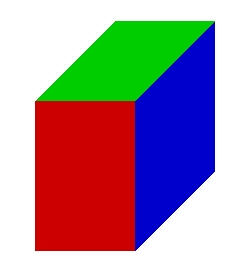# The block

The block has the dimensions of 5 cm, 10 cm, and 15 cm. Calculate the size of the wall diagonals of this block.

cm
cm
cmDid you find an error or inaccuracy? Feel free to write us. Thank you!Tips to related online calculators
Pythagorean theorem is the base for the right triangle calculator.

#### You need to know the following knowledge to solve this word math problem:

We encourage you to watch this tutorial video on this math problem: